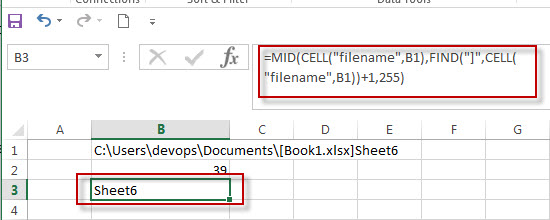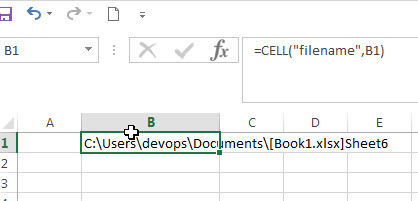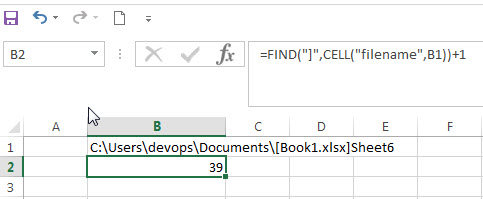## Get the Current Worksheet Name only

If you want to get the current worksheet name only in excel, you can use a combination of the MID function, the CELL function and FIND function. You can use the below generic formula:

`=MID(CELL("filename",B1),FIND("]",CELL("filename",B1))+1,255)`The below explains how the above excel formula works:

The CELL function will get the full information of the current worksheet contain the full file name and its path.

=CELL(“filename”,B1)The FIND function will locate the position of the right bracket symbol in the result returned by CELL function.

So the starting position of the filename of the current workbook can be got from the below formula:

`=FIND("]",CELL("filename",B1))+1`The MID function will extract the worksheet name from a text string returned by CELL function at the position that returned by the FIND function.

### Related Functions

• Excel Find function
The Excel FIND function returns the position of the first text string (sub string) from the first character of the second text string.The FIND function is a build-in function in Microsoft Excel and it is categorized as a Text Function.The syntax of the FIND function is as below:= FIND  (find_text, within_text,[start_num])…
• Excel MID function
The Excel MID function returns a substring from a text string at the position that you specify.The syntax of the MID function is as below:= MID (text, start_num, num_chars)…
• Excel CELL function
The Excel CELL function returns information about the formatting, location, size, or contents of a cell.The syntax of the CELL function is as below:= CELL (info_type,[reference])…